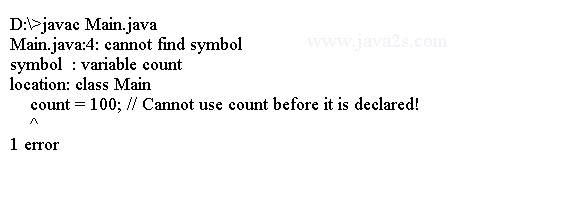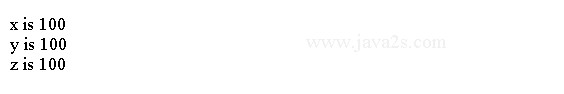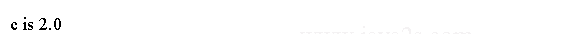# Java Tutorial - Java Variables

A variable is defined by an identifier, a type, and an optional initializer. The variables also have a scope(visibility / lifetime).

## Java variable type

In Java, all variables must be declared before they can be used. The basic form of a variable declaration is shown here:

```type identifier [ = value][, identifier [= value] ...] ;
```

There are three parts in variable definition:

• `type` could be `int` or `float`.
• `identifier` is the variable's name.
• Initialization includes an equal sign and a value.

To declare more than one variable of the specified type, use a comma-separated list.

```int a, b, c; // declares three ints, a, b, and c.
int d = 3, e, f = 5; // declares three more ints, initializing d and f.
```

The following variables are defined and initialized in one expression.

```public class Main {
public static void main(String[] argv) {
byte z = 2; // initializes z.
double pi = 3.14; // declares an approximation of pi.
char x = 'x'; // the variable x has the value 'x'.
}
}
```

Variable cannot be used prior to its declaration.

```public class Main {
public static void main(String[] argv) {

count = 100; // Cannot use count before it is declared!
int count;
}
}
```

Compiling the code above generates the following error message:## Assignment Operator

The assignment operator is the single equal sign, =. It has this general form:

```var = expression;
```

type of `var` must be compatible with the type of expression. The assignment operator allows you to create a chain of assignments.

```
public class Main {
public static void main(String[] argv) {
int x, y, z;
x = y = z = 100; // set x, y, and z to 100
System.out.println("x is " + x);
System.out.println("y is " + y);
System.out.println("z is " + z);
}
}
```

The output:## Dynamic Initialization

Java allows variables to be initialized dynamically. In the following code the `Math.sqrt` returns the square root of `2 * 2` and assigns the result to `c` directly.

```public class Main {
public static void main(String args[]) {

// c is dynamically initialized
double c = Math.sqrt(2 * 2);

System.out.println("c is " + c);
}
}
```

The output from the code above is